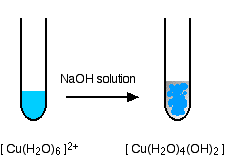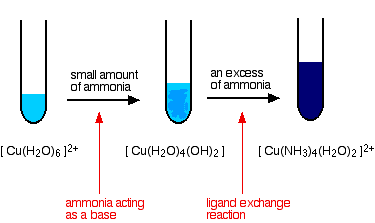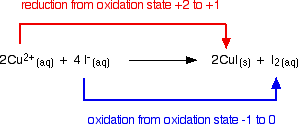# Chemistry of Copper

$$\newcommand{\vecs}{\overset { \rightharpoonup} {\mathbf{#1}} }$$ $$\newcommand{\vecd}{\overset{-\!-\!\rightharpoonup}{\vphantom{a}\smash {#1}}}$$$$\newcommand{\id}{\mathrm{id}}$$ $$\newcommand{\Span}{\mathrm{span}}$$ $$\newcommand{\kernel}{\mathrm{null}\,}$$ $$\newcommand{\range}{\mathrm{range}\,}$$ $$\newcommand{\RealPart}{\mathrm{Re}}$$ $$\newcommand{\ImaginaryPart}{\mathrm{Im}}$$ $$\newcommand{\Argument}{\mathrm{Arg}}$$ $$\newcommand{\norm}{\| #1 \|}$$ $$\newcommand{\inner}{\langle #1, #2 \rangle}$$ $$\newcommand{\Span}{\mathrm{span}}$$ $$\newcommand{\id}{\mathrm{id}}$$ $$\newcommand{\Span}{\mathrm{span}}$$ $$\newcommand{\kernel}{\mathrm{null}\,}$$ $$\newcommand{\range}{\mathrm{range}\,}$$ $$\newcommand{\RealPart}{\mathrm{Re}}$$ $$\newcommand{\ImaginaryPart}{\mathrm{Im}}$$ $$\newcommand{\Argument}{\mathrm{Arg}}$$ $$\newcommand{\norm}{\| #1 \|}$$ $$\newcommand{\inner}{\langle #1, #2 \rangle}$$ $$\newcommand{\Span}{\mathrm{span}}$$$$\newcommand{\AA}{\unicode[.8,0]{x212B}}$$

Copper occupies the same family of the periodic table as silver and gold, since they each have one s-orbital electron on top of a filled electron shell which forms metallic bonds. This similarity in electron structure makes them similar in many characteristics. All have very high thermal and electrical conductivity, and all are malleable metals. Among pure metals at room temperature, copper has the second highest electrical and thermal conductivity, after silver.

## Introduction

The use of Copper dates back far into history. Copper beads have been found in what is now modern Iraq, dating back to 9000 BC. The metal is relatively easy to mine and refine, contributing to its early and widespread use. Being soft, however, it is unsuitable for making reliable tools and weapons. Early metalsmiths as far back as 3000 BC learned to combine copper with other metals to produce more durable alloys. Brass (copper and zinc) and bronze (copper and tin) are two examples. The symbol and name for copper are from the Latin cuprum, which literally means "from the island of Cyprus", an early source of copper ore.

Before 1982 U.S. pennies were pure copper. Now they are mostly zinc with a thin shell of copper. Most copper that is mined today is refined and drawn into wire for use in the electrical industries. A significant portion is also used in manufacturing water pipe. Copper, of course, has a characteristic color which most people recognize. It is one of the best electrical conductors and resists corrosion from most acids (except nitric and hot concentrated sulfuric). When exposed to the elements for a period of time it develops a greenish coating or patina which is copper(II) carbonate, a protective coating that prevents further wear.

## Occurrence and extraction of Copper

Copper occurs both in combined state and free state and also in many ores. The important ores of copper are copper pyrites ($$CuFeS_2$$), cuprite and copper glance. The copper ores are mostly found in the north of India. The extraction of copper also involves many steps. The ore used for extraction is copper pyrites, which is crushed, concentrated and then heated in the presence of air. During heating the moisture gets expelled and the copper pyrites gets converted to ferrous sulfide and cuprous sulfide.

$2CuFeS_2 + O_2 \rightarrow Cu_2S + 2FeS + SO_2$

Blast furnace is used to heat the mixture of roasted ore, powdered coke and sand. In the blast furnace oxidation reactions takes place. Ferrous sulfide forms ferrous oxide which combines with silica and forms the slag ($$FeSiO_2$$).

$2FeS + 3O_2 \rightarrow 2FeO + 2SO_2$

$FeO + SiO_2 \rightarrow FeSiO_3$

Cuprous sulfide forms cuprous oxide which is partially converted to cuprous sulfide.

$Cu_2S + 3 O_2 \rightarrow 2Cu_2O + 2SO_2$

$Cu_2O + FeS \rightarrow Cu_2S + FeO$

This cuprous sulfide contains some amount of ferrous sulfide and this is called matte. Matte is removed from the base outlet of blast furnace. The removed matte is shifted into Bessemer converter which is lined inside with magnesium oxide. This converter has pipes through which hot air and $$SiO_2$$ are sent in. In this converter $$Cu_2S$$ converts to $$Cu_2O$$ and $$FeS$$ converts to $$FeO$$. The ferrous oxide forms slag with $$SiO_2$$. The cuprous oxide formed reacts with Cu2S and forms copper.

$2Cu_2O + Cu_2S \rightarrow 6Cu + SO_2$

The copper thus formed is to be purified by electrolysis.

## Reactions of copper(II) ions in solution

The simplest ion that copper forms in solution is the typical blue hexaaquacopper(II) ion - [Cu(H2O)6]2+.

### Reactions of hexaaquacopper(II) ions with Hydroxide Ions

Hydroxide ions (from, say, sodium hydroxide solution) remove hydrogen ions from the water ligands attached to the copper ion. Once a hydrogen ion has been removed from two of the water molecules, you are left with a complex with no charge - a neutral complex. This is insoluble in water and a precipitate is formed.The color coding is to show that this is not a ligand exchange reaction. The oxygens which were originally attached to the copper are still attached in the neutral complex.

In the test-tube, the color change is:### Reactions of hexaaquacopper(II) ions with ammonia solution

The ammonia acts as both a base and a ligand. With a small amount of ammonia, hydrogen ions are pulled off the hexaaqua ion exactly as in the hydroxide ion case to give the same neutral complex.

$[Cu(H_2O)_6]^{2+} + 2NH_3 \rightarrow [Cu(H_2O)_4(OH)_2] + 2NH_4^+$

That precipitate dissolves if you add an excess of ammonia. The ammonia replaces water as a ligand to give tetraamminediaquacopper(II) ions. Notice that only 4 of the 6 water molecules are replaced.

$[Cu(H_2O)_6]^{2+} + 4NH_3 \rightarrow [Cu(NH_3)_4(H_2O)_2]^{2+} + H_2O$

Note

You might wonder why this second equation is given starting from the original hexaaqua ion rather than the neutral complex. Explaining why the precipitate redissolves is quite complicated. You will find the explanation in full on the page about the reactions between hexaaqua ions and ammonia solution.

The color changes are:### The reaction of hexaaquacopper(II) ions with carbonate ions

You simply get a precipitate of what you can think of as copper(II) carbonate.

$Cu^{2+} + CO_3^{2-} \rightarrow CuCO_3(s)$## A ligand exchange reaction involving chloride ions

If you add concentrated hydrochloric acid to a solution containing hexaaquacopper(II) ions, the six water molecules are replaced by four chloride ions.The reaction taking place is reversible.

$Cu(H_2O)_6]^{2+} + 4Cl^- \rightleftharpoons [CuCl_4]^{2-} + 6H_2O$

Because the reaction is reversible, you get a mixture of colors due to both of the complex ions. The color of the tetrachlorocuprate(II) ion may also be described as olive-green or yellow. If you add water to the green solution, it returns to the blue color.

## The reaction of hexaaquacopper(II) ions with iodide ions

Copper(II) ions oxidize iodide ions to molecular iodine, and in the process are themselves reduced to copper(I) iodide.The initial mucky brown mixture separates into an off-white precipitate of copper(I) iodide under an iodine solution.### Using this reaction to find the concentration of copper(II) ions in solution

If you pipette a known volume of a solution containing copper(II) ions into a flask, and then add an excess of potassium iodide solution, you get the reaction we have just described.

$2Cu^{2+} + 4I^- \rightarrow 2CuI(s) +I_2 (aq)$

You can find the amount of iodine liberated by titration with sodium thiosulphate solution.

$2S_2O_3^{2-} (aq) + I_2 (aq) \rightarrow S_4O_6^{2-} (aq) + 2I^- (aq)$

As the sodium thiosulfate solution is run in from a burette, the color of the iodine fades. When it is almost all gone, you add some starch solution. This reacts reversibly with iodine to give a deep blue starch-iodine complex which is much easier to see.

You add the last few drops of the sodium thiosulfate solution slowly until the blue color disappears. If you trace the reacting proportions through the two equations, you will find that for every 2 moles of copper(II) ions you had to start with, you need 2 moles of sodium thiosulfate solution. If you know the concentration of the sodium thiosulfate solution, it is easy to calculate the concentration of the copper(II) ions.

## Some essential copper(I) chemistry

### The disproportionation of copper(I) ions in solution

Copper(I) chemistry is limited by a reaction which occurs involving simple copper(I) ions in solution. This is a good example of disproportionation - a reaction in which something oxidises and reduces itself. Copper(I) ions in solution disproportionate to give copper(II) ions and a precipitate of copper. The reaction is:Any attempt to produce a simple copper(I) compound in solution results in this happening. For example, if you react copper(I) oxide with hot dilute sulfuric acid, you might expect to get a solution of copper(I) sulfate and water produced. In fact you get a brown precipitate of copper and a blue solution of copper(II) sulfate because of the disproportionation reaction.

$Cu_2O + H_2SO_4 \rightarrow Cu + CuSO_4 + H_2O$

## Stabilizing the copper(I) oxidation state

We've already seen that copper(I) iodide is produced as an off-white precipitate if you add potassium iodide solution to a solution containing copper(II) ions. The copper(I) iodide is virtually insoluble in water, and so the disproportionation reaction does not happen. Similarly copper(I) chloride can be produced as a white precipitate (reaction described below). Provided this is separated from the solution and dried as quickly as possible, it remains white. In contact with water, though, it slowly turns blue as copper(II) ions are formed. The disproportionation reaction only occurs with simple copper(I) ions in solution.

Forming copper(I) complexes (other than the one with water as a ligand) also stabilizes the copper(I) oxidation state. For example, both [Cu(NH3)2]+ and [CuCl2]- are copper(I) complexes which do not disproportionate. The chlorine-containing complex is formed if copper(I) oxide is dissolved in concentrated hydrochloric acid. You can think of this happening in two stages. First, you get copper(I) chloride formed:

$Cu_2O_{(s)} + 2HCl_{(aq)} \rightarrow 2CuCl_{(s)} + H_2O_{(l)}$

But in the presence of excess chloride ions from the HCl, this reacts to give a stable, soluble copper(I) complex.

$CuCl_{(s)} + Cl^-_{(aq)} \rightarrow [CuCl_2]^-_{(aq)}$

You can get the white precipitate of copper(I) chloride (mentioned above) by adding water to this solution. This reverses the last reaction by stripping off the extra chloride ion.